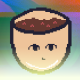# SGDK. Move the sprite across the screen.

This tutorial is a continuation of the SGDK article. Create an image.

## Import the sprite.

Download the sprite mug with MEGA. And throw it in the res folder, in the directory of your project.

Open the previous project, and in resources.res, add the following line.

``SPRITE spr_cup "cup.png" 8 8 BEST``

The syntax here is as follows

``type name path_to_file width_in_tiles height_in_tiles compression_type``

That is, to the sprite cup.png refer to by the name spr_cup.

The size of the sprite is 64×64 pixels, or 8×8 in tiles (1 tile = 8×8 pixels)

Compression chose the best BEST.

As with images, all resource information is in recomp.txt located in SGDK/bin

## Write the code.

Copy the following code.

``````#include <genesis.h>
#include "resources.h"

Sprite* cup_obj;
s16 x = 0;
s16 y = 0;

int main()
{
VDP_drawImage(BG_A, &img, 0, 0);
SPR_init();
VDP_setPalette(PAL3, spr_cup.palette->data);
cup_obj = SPR_addSprite(&spr_cup, x, y, TILE_ATTR(PAL3, 0, FALSE, FALSE));
while(1)
{
SPR_update();
SYS_doVBlankProcess();
}
return (0);
}</genesis.h>``````

Now, let’s break it down.

``Sprite* cup_obj;``

The Sprite type, as the name implies, stores sprites. The asterisk makes it clear that we are creating a pointer *, therefore, we will pass it by link &. In this variable, we will store the sprite.

``````s16 x = 0;
s16 y = 0;``````

Created variables in which we will store the coordinates of the sprite.

Type s16 is a 16-bit number with a sign (+ or -), in other words signed 16. C s8 and s32 are the same principle.

There is also a type u1616 bit number without a sign, in other words unsigned 16. C u8 and u32 are the same principle.

``SPR_init();``

SPR_init – initializes the sprite engine (allocates space in VRAM for sprites)

VRAM (Video Random Access Memory) – RAM capacity of 64 KB,storing tiles of the image.

``VDP_setPalette(PAL3, spr_cup.palette->data);``

In this line, we set the colors in the 4th palette (countdown starts from zero),colors taken from the sprite of the mug.

Sega Genesis supports 4 palettes of 16 colors (PAL0-PAL3), and stores them in CRAM.

``cup_obj = SPR_addSprite(&spr_cup, x, y, TILE_ATTR(PAL3, 0, FALSE, FALSE));``

SPR_addSprite adds a sprite to the screen, the syntax is as follows.

``SPR_addSprite(sprite, x, y, tile_attributes);``

Consider the tile_attributes.

``TILE_ATTR(palette, priority, flip_vertical, flip_horizontal)``
• Palette – Specify the palette that the tiles will use (in our case, the sprite)
• Priority – sets the priority of the sprite (tile), i.e. a sprite with a smaller number, will overlap the sprite with a large one.
• the rest, the title implies.

In our case

``TILE_ATTR(PAL3, 0, FALSE, FALSE)``
• We used a 4th palette, in which just above, we placed a palette taken from the sprite of the mug.
• The priority is maximum.
• On the x-axis, we will notflip the sprite.
• Also on the y-axis.

So we created a sprite, and put cup_obj in thevariable.

``SPR_update();``

Updates and displays sprites on the screen.

``SYS_doVBlankProcess();``

SYS_doVBlankProcess – does all the behind-the-scenes processing, you need it when you are using sprites, music, joystick. In general, it is always needed.

Now, compile and run. You should get the following.

Okay, it’s left to make this cup move. Let’s make it start from the boundaries of the screen.

## Move the mug.

Add variables responsible for the speed and size of the sprite.

``````s16 x_spd = 3;
s16 y_spd = 3;
u16 cup_width = 64;
u16 cup_height = 64;``````

In the whileloop , add the following code

``````x += x_spd;
y += y_spd;
if(x > 320-cup_width || x < 0)
x_spd *= -1;
if(y > 240-cup_height || y < 0)
y_spd *= -1;
SPR_setPosition(cup_obj, x, y);``````

The sprite is constantly moving at a given speed.

``x += x_spd;  y += y_spd;``

If it has gone beyond the boundaries of the screen, then change the speed to the opposite.

``if(x > 320-cup_width || x < 0)  x_spd *= -1;  if(y > 240-cup_height || y < 0)  y_spd *= -1;``

And set the sprite position to x,y.

``SPR_setPosition(cup_obj, x, y);``

As a result, we got a wall-repelled cup sprite .

## Final result.#### bolon667

View all posts by bolon667 →